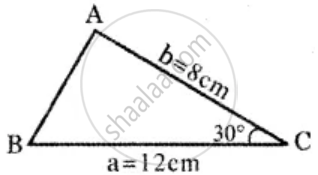Tamil Nadu Board of Secondary EducationHSC Arts Class 11th

# In a ∆ABC, if a = 12 cm, b = 8 cm and C = 30°, then show that its area is 24 sq.cm - Mathematics

Sum

In a ∆ABC, if a = 12 cm, b = 8 cm and C = 30°, then show that its area is 24 sq.cm

#### SolutionIn ∆ABC Given

a = 12 cm,

b = 8 cm,

C = 30°

Area ∆ = 1/2 ab sin C

= 1/2 xx 12 xx 8 xx sin 30^circ

= 6 xx 8 xx 1/2

= 6 × 4

= 24 sq.cm

Concept: Application to Triangle
Is there an error in this question or solution?

Share